# Monarch Digital - CPM Calculator

Definition:

CPM: Cost-Per-Mille, a standard cost calculation for digital ad campaigns where the client pays a set amount per 1,000 impressions. (The “mille” being latin for thousand).

Application:

While the actual calculation is relatively simple, (CPM x impressions = total spend) Monarch provides a method to calculate any of the three values, to help find the best impact for their money.

An advertiser may say, “I’ve got \$5,000.00 to spend, how many impressions will that get me?” Or they may ask the opposite – I want 10,000,000 impressions, how much would that cost?”

The CPM calculator is set up to help me get that answer as quickly as possible.

Access:

First, a superuser or admin will need to select the Digital or Enterprise business profile from the administrator section of the site:

Step 1: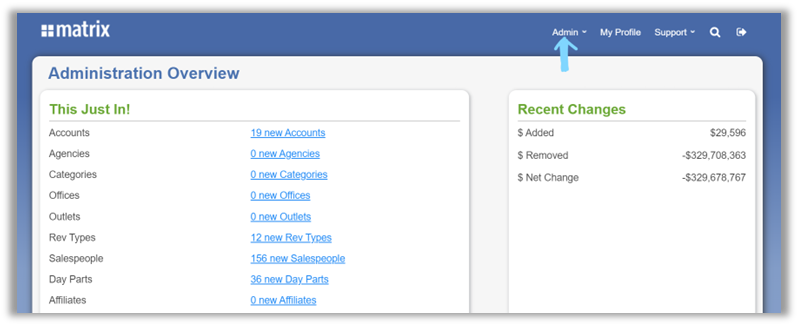Step 2: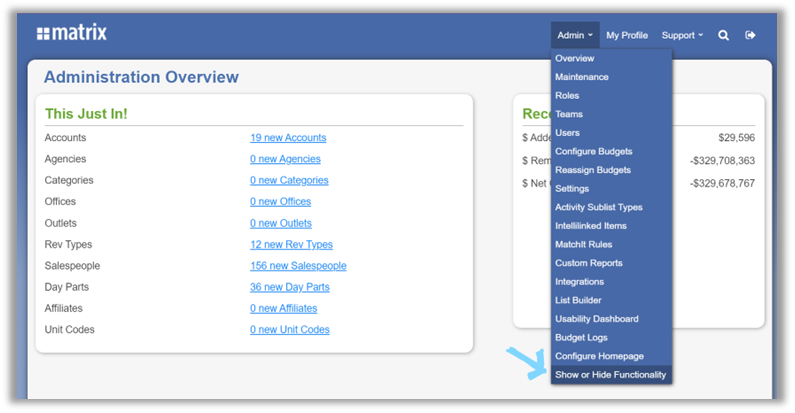Step 3:NOTE: When selecting the Enterprise Business Profile, there is an extra step to activate the CPM Calculator:Once the Digital or Enterprise business profile has been selected, users can log into the Monarch site and see the CPM Calculator link in the bottom-left corner of the site, above the “Live Chat” link: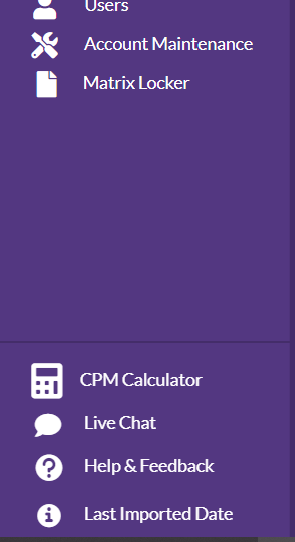Using the CPM Calculator

Clicking the link reveals the CPM calculator on the right side of the web page: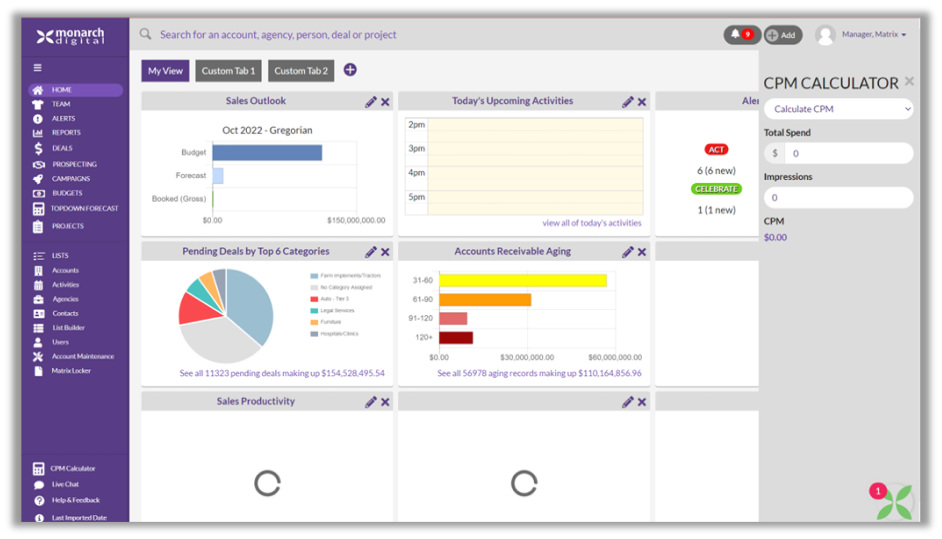This diagram shows the ways in which I can interact with the calculator:The drop-down has three options:Calculate CPM:

I can input TOTAL SPEND and TOTAL IMPRESSIONS. As I input the numbers in the second field, the calculator will show the result under Total CPM. In this example, I’ve input total spend as \$5000, total impressions at 1,000,000, resulting in a CPM of \$5.00.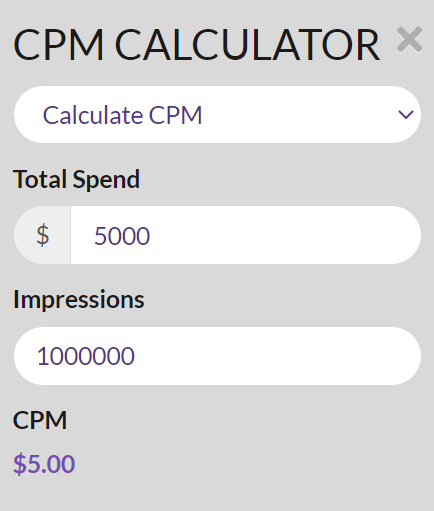Calculate Impressions:

I can input TOTAL CPM and TOTAL SPEND. As I input the numbers in the second field, the calculator will show the result under Total impressions. In this example, I’ve input \$5.00 CPM, total spend of \$10,000, resulting in a total of 2,000,000 impressions.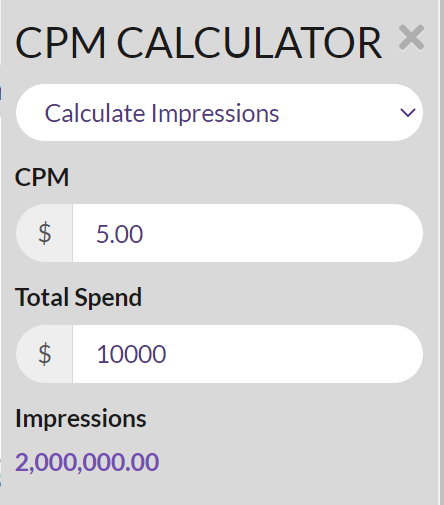Calculate Total Spend: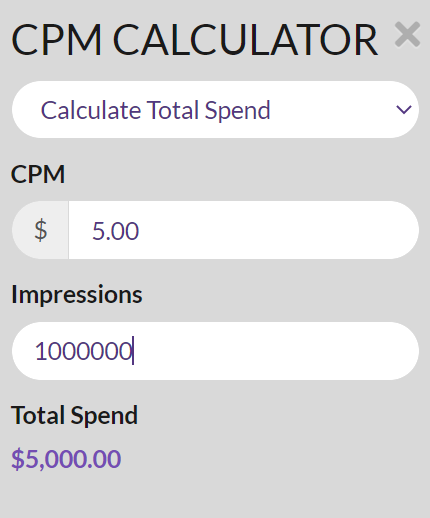I can input TOTAL CPM and TOTAL IMPRESSIONS. As I input the numbers in the second field, the calculator will show the result under Total Spend. In this case, I’ve input CPM of \$5.00, total impressions of 1,000,000, resulting in a total spend of \$5,000.00.

USE CASES:

As a salesperson, when putting together a deal, this calculator will decrease the time it takes to properly fill out totals for the three fields (impressions, total spend, CPM).